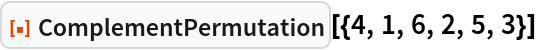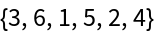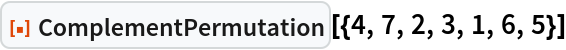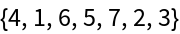Function Repository Resource:

# ComplementPermutation

Get the complement of a permutation

Contributed by: Motoharu Ito
 ResourceFunction["ComplementPermutation"][perm] returns the complement of the permutation perm.

## Details

The complement permutation c(p) of the permutation p=p1p2…pn is the permutation p1'p2'…pn' where pk'=n+1-pk.

## Examples

### Basic Examples (1)

Find the complement of a permutation:

 In:=Out=### Scope (1)

The complement of an alternating permutation is a reverse alternating permutation (a descent followed by alternating ascents and descents):

 In:=Out=Motoharu Ito

## Version History

• 1.0.0 – 28 November 2022